# Electronics Engineering - Online Test

Q1. Circuit turn-off time of an SCR is defined as the time
Explaination / Solution:
No Explaination.

Q2. Solution of the variables x1 and x2 for the following equations is to be obtained by employing the Newton-Raphson iterative method.
equation (i)    10x2 sinx1 - 0.8 = 0
equation (ii)    10x2 - 10x2 cosx1 - 0.6 = 0
Assuming the initial valued x1 = 0.0 and x2 = 1.0, the jacobian matrix is
Explaination / Solution: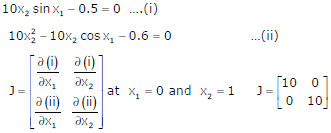Q3. A dual trace oscilloscope is set to operate in the Alternate mode. The control input of the multiplexer used in the y-circuit is fed with a signal having a frequency equal to
Explaination / Solution:
No Explaination.

Q4. The output Y of the logic circuit given below isExplaination / Solution: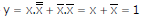Q5.
The bridge circuit shown in the figure below is used for the measurement of an unknown element ZX. The bridge circuit is best suited when ZX is a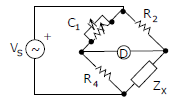Explaination / Solution:

Q6. The r.m.s value of the current i(t) in the circuit shown below is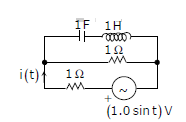Explaination / Solution: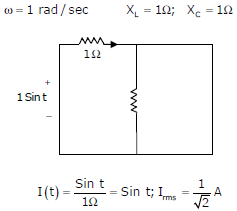Q7. What is the minimum number of gates required to implement the Boolean function (AB + C) if we have to use only 2-input NOR gates?
Explaination / Solution:
No Explaination.

Q8.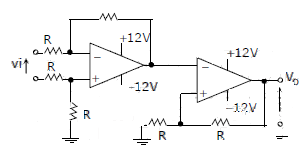The CORRECT transfer characteristic is

Explaination / Solution:

It is a Schmitt trigger and phase shift is zero.

Q9. Given a vector field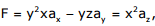the line integral ∫ F.dl evaluated along a segment on the x-axis from x=1 to x=2 is
Explaination / Solution:
No Explaination.

Q10. If R(τ) is the auto correlation function of a real, wide-sense stationary random process, then which of the following is NOT true
Explaination / Solution:
No Explaination.